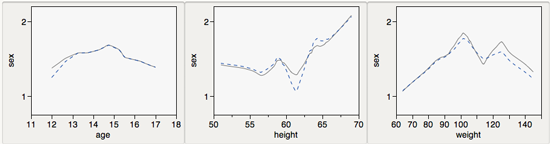Choose Language Hide Translation BarStaffJoined:

Jun 9, 2012

Marginal Model Plot Add-in for JMP

If you attended this year’s Discovery conference, you may recall Dr. Sheather’s enlightening presentation on marginal model plots. Now, you can easily create marginal model plots in JMP with the Marginal Model Plot Add-in.

Marginal model plots are a great addition to your model assessment toolbox — particularly in cases where well-known techniques like residual analysis are found lacking in some respect. For example, consider a setting where you are trying to predict a binary response. In this case, residual plots are not very helpful in diagnosing model fit, but marginal model plots offer a way to visually assess differences between the data and the predictions made by the model.

A given predictor’s marginal model plot shows two curves on the same set of axes:

• a locally-smoothed curve of the response vs. the predictor, and
• a locally-smoothed curve of the fitted values vs. the predictor.
• In cases where the response or the predictor is categorical, the add-in replaces the categories with numeric values so that smoothing can be performed.

If the model fits the data well, this pair of curves will be similar—for any predictor we choose. Conversely, if we observe any plot having poorly-matched curves, this suggests that model fit is lacking in some respect.

Consider an example in which we have built a model to predict the gender of a child (females=1, males=2), given his or her age, height and weight. In the marginal model plots below, the general shape of the curves in each pair is similar, but in each plot there is some region where the smoothed curve for the actuals vs. the predictor (gray solid line) diverges from the graph of the smoothed curve of the fits vs. the predictor (blue dashed line.) This tells us that while our model has us off to a good start, it does have some shortcomings. For example, 12-year-olds are overclassified as females, as are children over 120 pounds.For more information on the use of marginal model plots, please see Cook and Weisburg (1977).

I’ve placed the Marginal Model Plot Add-in in the “Utility” subsection of the “Add-ins” section of the JMP File Exchange. Be sure to check it out soon! (Download of add-in requires a free SAS profile.)

1 Comment
Community Member

Elizabeth Kolodziej wrote:

This is exciting! I was wondering whether there exists an easy way to create marginal model plots in SAS 9.3, or whether they might add a marginal model plot function to the next version of SAS, as well as JMP. Thanks!

Article Tags
Labels